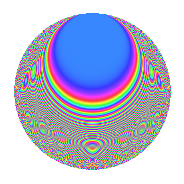# Properties

 Label 112.2.vLevel 112 Weight 2 Character orbit v Rep. character $$\chi_{112}(3,\cdot)$$ Character field $$\Q(\zeta_{12})$$ Dimension 56 Newform subspaces 1 Sturm bound 32 Trace bound 0

# Related objects

## Defining parameters

 Level: $$N$$ = $$112 = 2^{4} \cdot 7$$ Weight: $$k$$ = $$2$$ Character orbit: $$[\chi]$$ = 112.v (of order $$12$$ and degree $$4$$) Character conductor: $$\operatorname{cond}(\chi)$$ = $$112$$ Character field: $$\Q(\zeta_{12})$$ Newform subspaces: $$1$$ Sturm bound: $$32$$ Trace bound: $$0$$

## Dimensions

The following table gives the dimensions of various subspaces of $$M_{2}(112, [\chi])$$.

Total New Old
Modular forms 72 72 0
Cusp forms 56 56 0
Eisenstein series 16 16 0

## Trace form

 $$56q - 2q^{2} - 6q^{3} - 4q^{4} - 6q^{5} - 8q^{7} + 4q^{8} + O(q^{10})$$ $$56q - 2q^{2} - 6q^{3} - 4q^{4} - 6q^{5} - 8q^{7} + 4q^{8} - 24q^{10} + 2q^{11} - 6q^{12} + 16q^{14} + 8q^{16} - 12q^{17} - 30q^{18} - 6q^{19} - 10q^{21} - 28q^{22} - 12q^{23} - 6q^{24} - 6q^{26} + 26q^{28} - 24q^{29} - 18q^{30} - 12q^{32} - 12q^{33} - 2q^{35} + 16q^{36} + 6q^{37} - 6q^{38} - 4q^{39} - 66q^{40} + 70q^{42} + 26q^{44} + 12q^{45} + 16q^{46} - 8q^{49} - 34q^{51} + 84q^{52} + 6q^{53} + 42q^{54} + 16q^{56} + 18q^{58} + 42q^{59} + 78q^{60} - 6q^{61} - 16q^{64} - 4q^{65} + 126q^{66} + 6q^{67} + 24q^{68} - 80q^{70} - 80q^{71} - 4q^{72} + 62q^{74} + 24q^{75} + 10q^{77} + 4q^{78} + 12q^{80} - 8q^{81} + 42q^{82} - 152q^{84} - 28q^{85} - 12q^{87} + 30q^{88} + 16q^{91} - 20q^{92} + 10q^{93} - 42q^{94} + 36q^{96} - 108q^{98} - 16q^{99} + O(q^{100})$$

## Decomposition of $$S_{2}^{\mathrm{new}}(112, [\chi])$$ into newform subspaces

Label Dim. $$A$$ Field CM Traces $q$-expansion
$$a_2$$ $$a_3$$ $$a_5$$ $$a_7$$
112.2.v.a $$56$$ $$0.894$$ None $$-2$$ $$-6$$ $$-6$$ $$-8$$

## Hecke Characteristic Polynomials

There are no characteristic polynomials of Hecke operators in the database